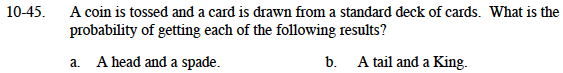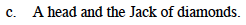### Home > MC1 > Chapter 10 > Lesson 10.1.4 > Problem10-45

10-45.$\frac{1}{2}\left( \frac{1}{4}\right)=\frac{1}{8}$

The probability of getting a head is one-half.
The probability of getting a spade is one-fourth.

The probability of getting a tail is one-half.
The probability of getting a King is one-thirteenth.Refer to parts (a) and (b) for help.